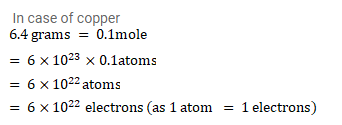# Assume that each atom in a copper wire contributes one free electron.Question:

Assume that each atom in a copper wire contributes one free electron. Estimate the number of free electrons in a copper wire having a mass of $6.4 \mathrm{~g}$ (take the atomic weight of copper to be $64 \mathrm{~g} / \mathrm{mol}$ ).

Solution: